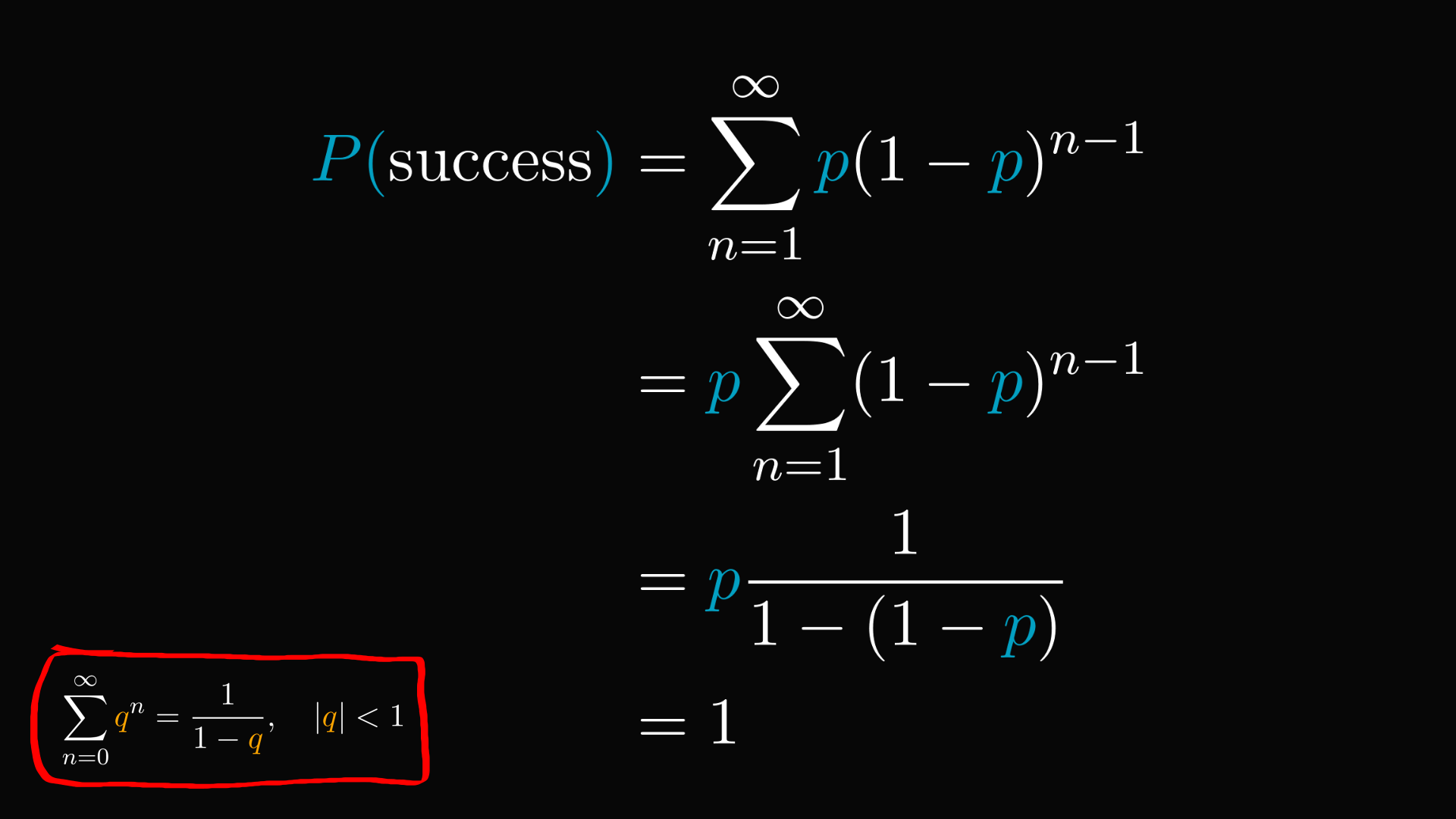# The Infinite Monkey Theorem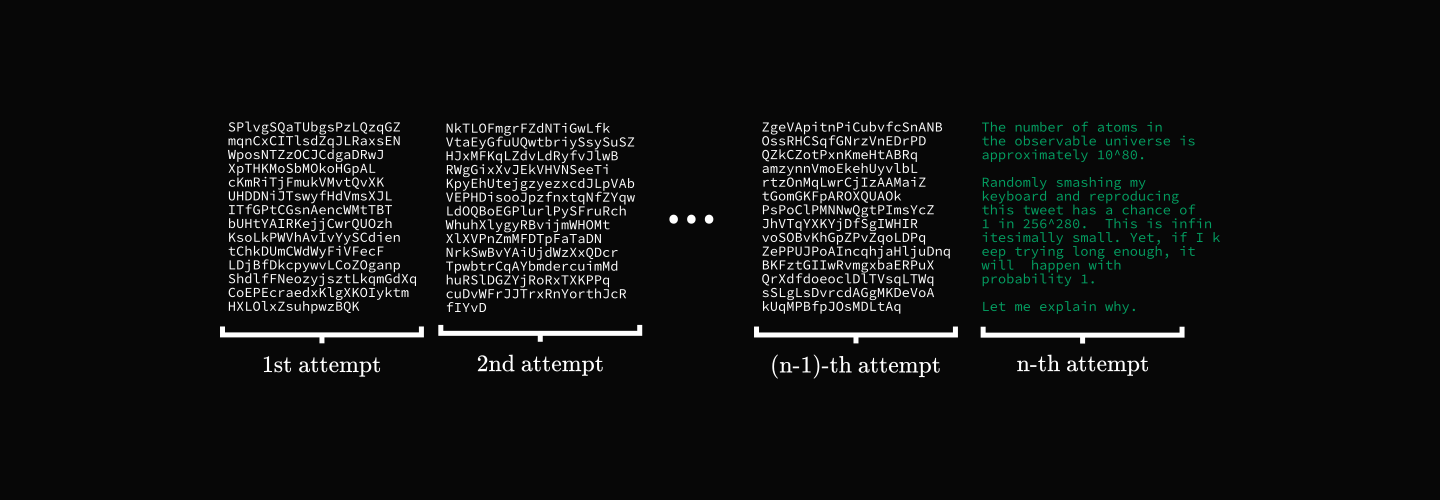The number of atoms in the observable universe is approximately $10^80$.

Randomly smashing my keyboard and reproducing this tweet has a chance of $1$ in $256^280$. This is infinitesimally small. Yet, if I keep trying long enough, it will happen with probability $1$.

Let me explain why.

(This post was originally posted in a Twitter thread, which why I am talking about tweets here.)First things first. If we generate a random string of $5$ characters, what is the probability of getting "hello"?

Assuming only ASCII characters, we have $256$ options in total.

Thus, each character has a $1/256$ probability of hitting the right one.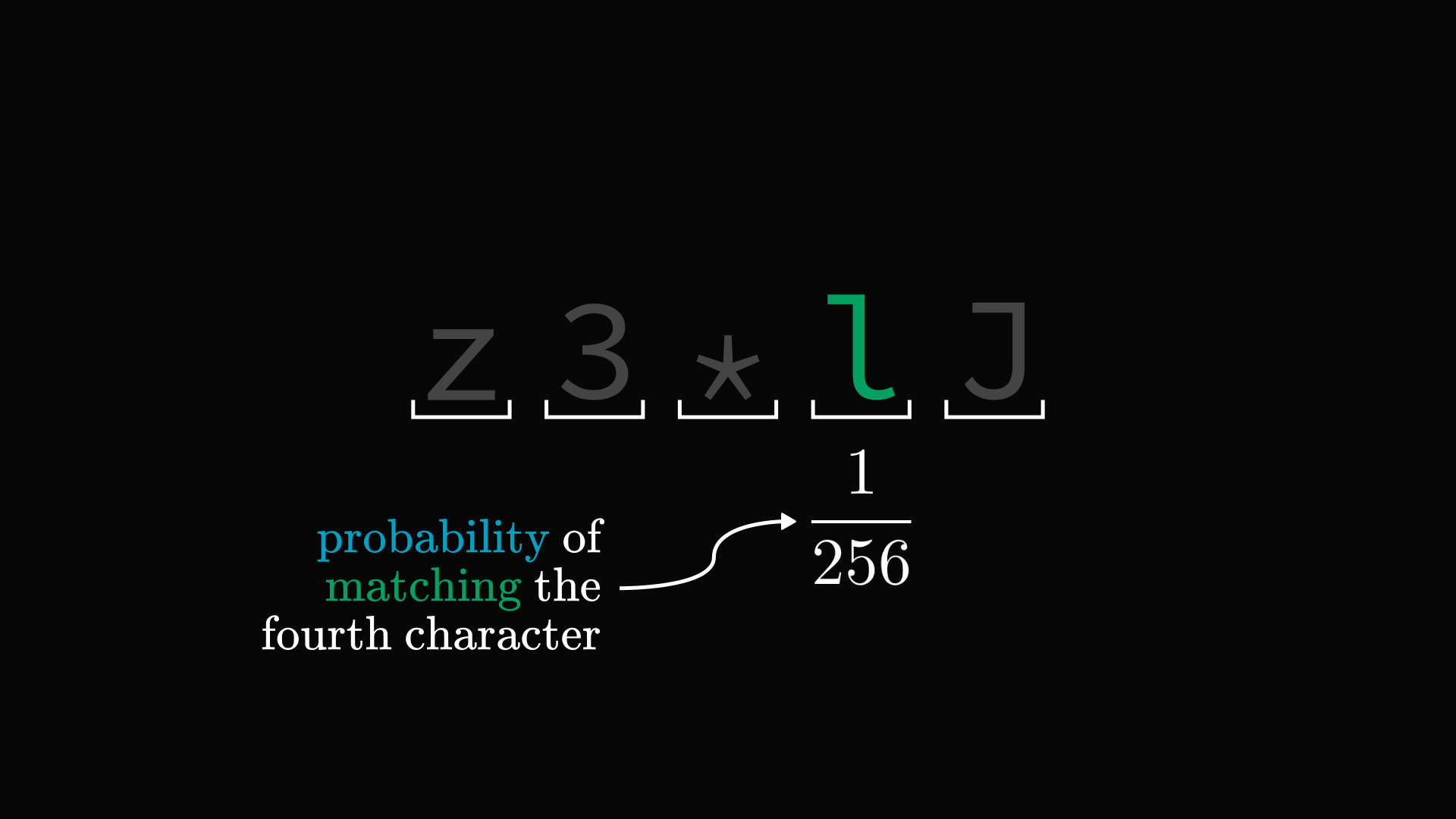If we are truly random in our selections, each choice is independent of the others.

Thus, hitting every character has a probability of $\frac{1}{256^5}$.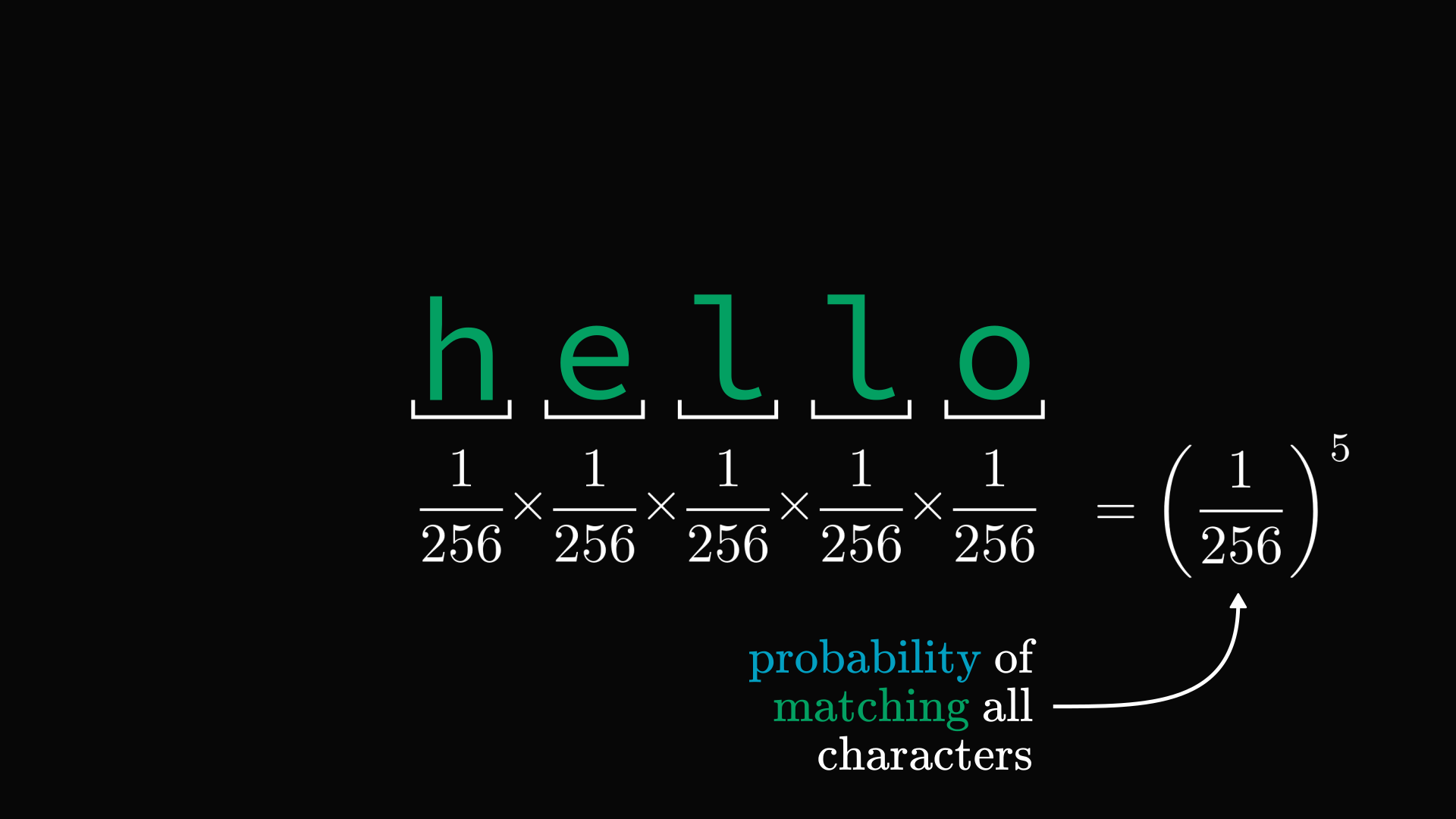Now imagine randomly generating a tweet, which is a $280$-character string.

Following the same logic, matching a given tweet has a probability of $p = \frac{1}{256^{280}}$.

It's beyond comprehension how small this is. Why then it is a certainty that I'll hit this if I keep trying?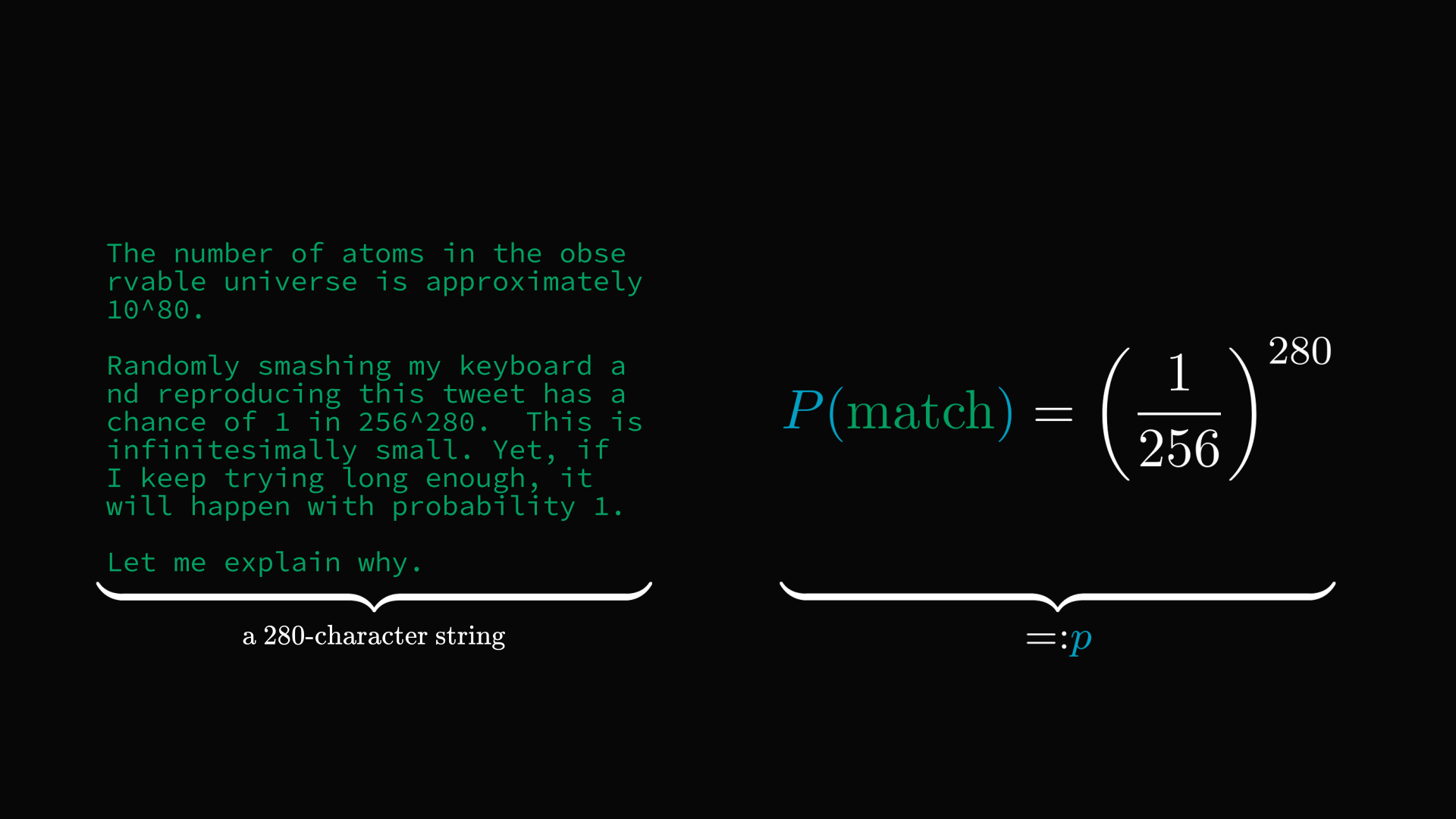Let's say I am randomly generating tweets one at a time.

What's the probability that I'll succeed in matching on the n-th attempt?

Success per attempt has a probability $p$, while failure has $(1-p)$. Thus, we can calculate the odds of $(n-1)$ failures and one success.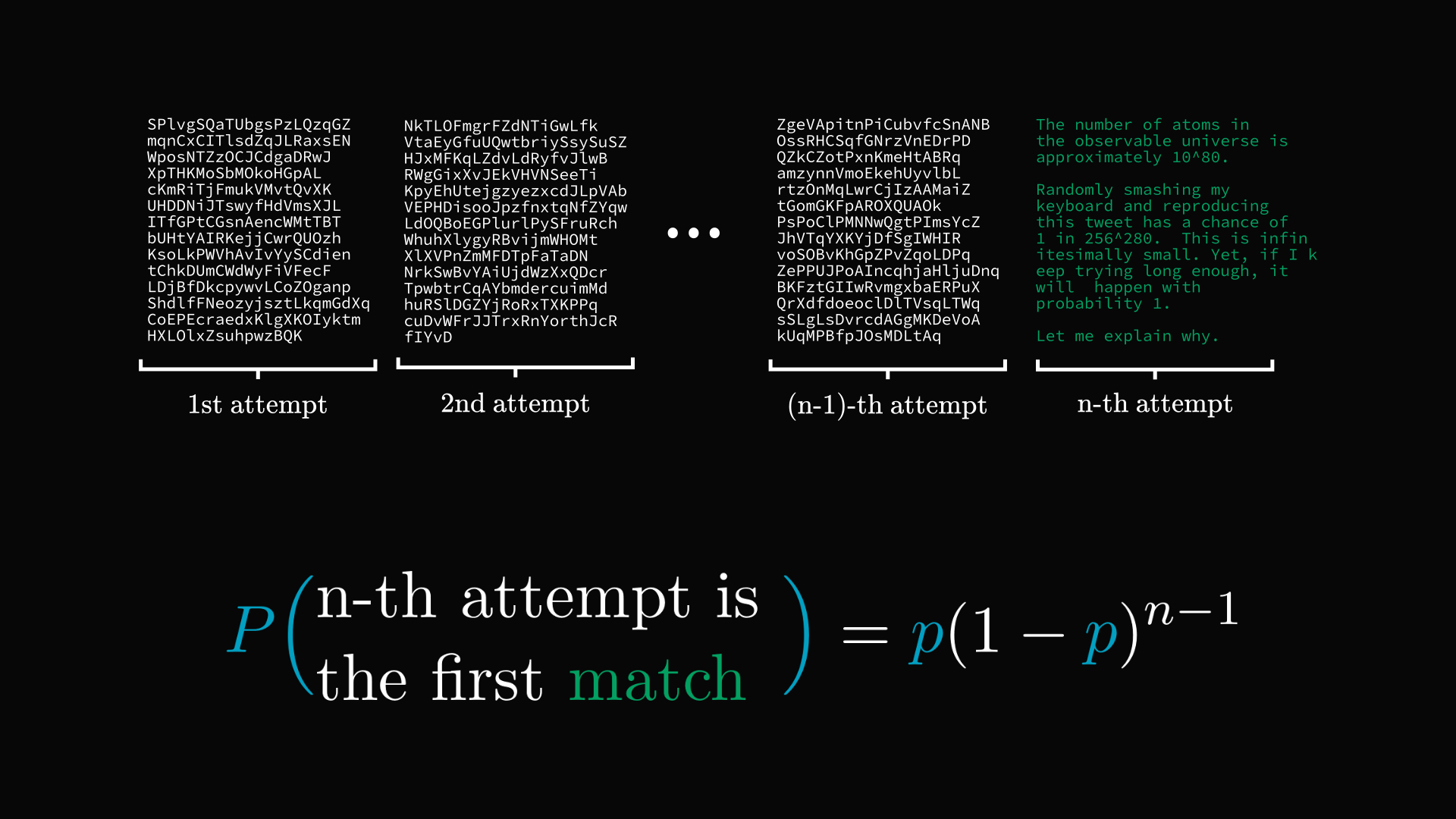What is the probability that I'll randomly reproduce my first tweet at some point?

Well, I can be successful either on the first attempt, or the second, or the third, and so on...

Thus, we can sum up the probabilities to get the chance of eventual success.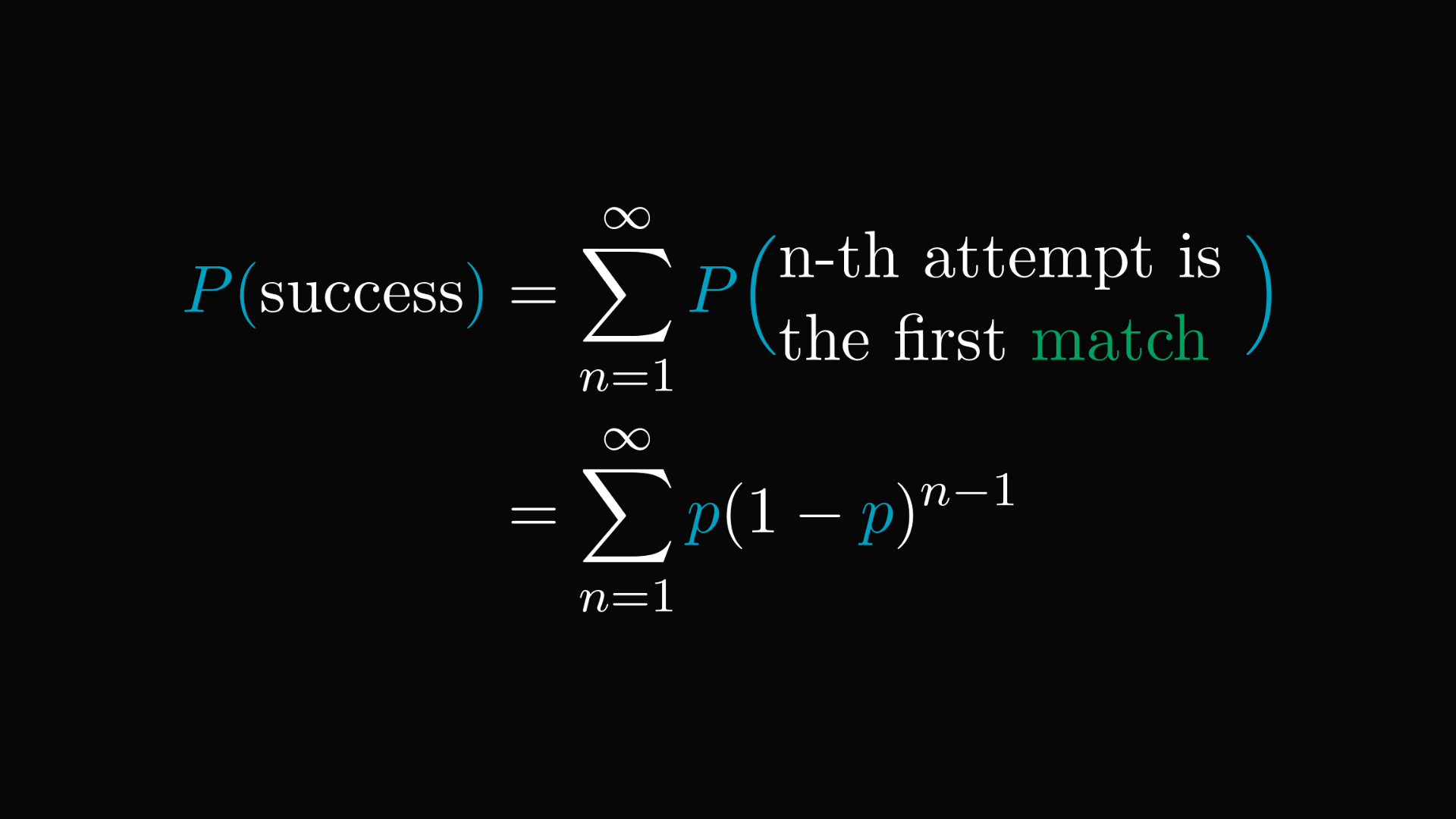Surprise: the probability of success is $1$. (We used the famous closed formula for the geometric series.)

This is why a monkey smashing a typewriter will eventually reproduce the complete works of Shakespeare.

How much you have to wait is a question for another day.